# Electrical Engineering Formulas (Most Important Equations)

Contents

## Formulas For Electrical Engineering

Electrical engineering is a branch that deals with the study, design, and implementation of various electrical equipment used in day-to-day life.

It covers a wide range of topics like; power systems, electrical machines, power electronics, computer science, signal processing, telecommunication, control system, artificial intelligence, and many more.

This branch of engineering is full of formulas and concepts (laws) used in many aspects like solving circuits and implementing different equipment to make human life more manageable.

The basic formulas commonly used in various electrical engineering subjects are listed below.

## Voltage

Voltage is defined as the electrical potential difference per unit charge between two points in the electric field. The unit of voltage is Volt (V).

(1)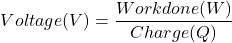From the above equation, the unit of voltage is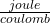## Current

Electric current is defined as a flow of charged particles (electrons and ions) moving through a conductor. It is also defined as the flow rate of electric charge through a conducting medium concerning time.

The unit of electric current is ampere (A). And the electric current is denoted mathematically by the symbol ‘I’ or ‘i’.

(2)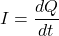## Resistance

Resistance or electrical resistance measures the opposition to current flow in an electrical circuit. Resistance is measured in ohms (Ω).

Resistance of any conducting material is directly proportional to length of material, and inversely proportional to the area of conductor.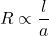(3)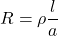Where,= proportionality constant (specific resistance or resistivity of conducting material)

According to the ohm’s law;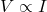(4)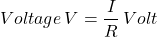Where, R = Resistance of conductor (Ω)

(5)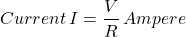(6)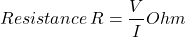## Electric Power

Power is the rate of energy supplied or consumed by an electric element with respect to time.

(7)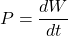### For DC System

(8)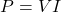(9)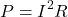### For Single-phase System

(10)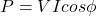(11)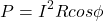(12)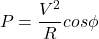### For Three-phase system

(13)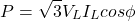(14)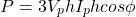(15)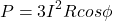(16)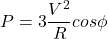## Power Factor

The power factor is a very important term in case of the AC system. It is defined as a ratio of working power absorb by the load to the apparent power flowing through the circuit.

(17)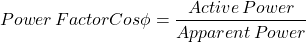The power factor dimensions less number in the closed interval of -1 to 1. When the load is resistive, power factor is near to 1 and when the load is reactive, power factor is near to -1.

## Frequency

Frequency is defined as the number of cycles per unit time. It is denoted as f and measured in Hertz (Hz). One hertz is equal to one cycle per second.

Generally, the frequency is 50 Hz or 60 Hz.

The time period is defined as the time required to produce one complete waveform cycle, denoted as T.

The frequency is inversely proportional to time period (T).

(18)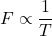## Wavelength

Wavelength is defined as a distance between consecutive corresponding points (two adjacent crests, or zero crossing).

It is defined as a ratio of velocity and frequency for sinusoidal waves.

(19)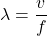## Capacitance

A capacitor stores electrical energy in an electric field when voltage is supplied. The effect of capacitors in electrical circuits is known as capacitance.

The electric charge Q accumulated in capacitor is directly proportional to the voltage developed across the capacitor.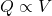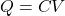(20)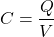The capacitance depends on the distance between two plates (d), area of plate (A), and permittivity of dielectric material.

(21)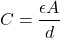## Inductor

An inductor stores electrical energy in the form of a magnetic field when electric current flows through it. Sometimes, an inductor is also known as coil, reactor, or chokes.

The unit of inductance is henry (H).

The inductance is defined by a ratio of magnetic flux linkage (фB), and the current passes through the inductor (I).

(22)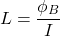## Electric Charge

Electric charge is a physical property of substance. When any matter is placed in an electromagnetic field, it will experience a force.

Electric charges can be positive (proton) and negative (electron), measured in coulomb and denoted as Q.

One coulomb is defined as the quantity of charge transferred in one second.

(23)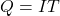## Electric Field

An electric field is a field or space around an electrically charged object where any other electrically charged object will experience a force.

An electric field is also known as electric field intensity or electric field strength, denoted by E.

Electric field is defined as a ratio of electrical force per test charge.

(24)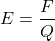For parallel plate capacitor, the voltage difference between two plates is expressed a work done on a test charge Q to move from the positive plate to negative plate.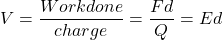(25)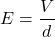## Electric Force

When a charged object enters the electric field of another charged object, it experiences a force as per Coulomb’s law.

As shown in the above figure, a positively charged object is placed in space. If both objects have the same polarity, objects repel each other. And if both objects have different polarities, objects attract each other.

According to coulomb’s law,

(26)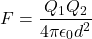According to coulomb’s law, the equation of electric field is;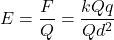(27)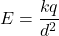## Electric Flux

According to gauss’s law, the equation of electric flux is;

(28)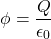## DC Machine

### Back EMF

(29)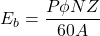### Losses in DC Machine

#### Copper loss

The copper losses occur due to current flowing through the windings. The copper loss is directly proportional to the square of current flowing through the winding, and is also known as I2R loss or ohmic loss.

Armature copper loss: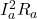Shunt field copper loss: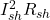Series field copper loss: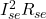Copper loss in interpole: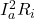Brush contact loss: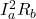#### Hysteresis Loss

Hysteresis loss occurs due to the reversal of magnetism of the armature core.

(30)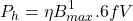#### Eddy Current Loss

The power loss that occurs due to eddy current flow is known as eddy current loss.

(31)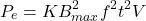### Transformer

#### EMF Equation

(32)#### Turns Ratio

(33)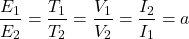#### Voltage Regulation

(34)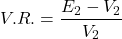### Induction Motor

#### Synchronous Speed

(35)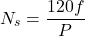#### Torque Equation

Developed Torque

(36)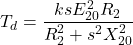Shaft Torque

(37)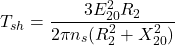#### Winding EMF

(38)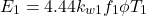(39)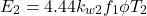Where,

Kw1, Kw2 = Winding factor of stator and rotor, respectively

T1, T2 = Number of turns in stator and rotor winding

Want To Learn Faster? 🎓
Get electrical articles delivered to your inbox every week.
No credit card required—it’s 100% free.